Ch 6. Advanced Beams Multimedia Engineering Mechanics CompositeBeams UnsymmetricBending
 Chapter 1. Stress/Strain 2. Torsion 3. Beam Shr/Moment 4. Beam Stresses 5. Beam Deflections 6. Beam-Advanced 7. Stress Analysis 8. Strain Analysis 9. Columns Appendix Basic Math Units Basic Equations Sections Material Properties Structural Shapes Beam Equations Search eBooks Dynamics Fluids Math Mechanics Statics Thermodynamics Author(s): Kurt Gramoll ©Kurt GramollMECHANICS - EXAMPLE Example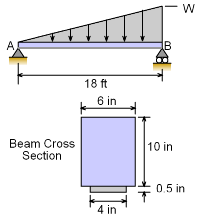Example Graphic Determine the safe uniformly varying load, Wo that can be applied to a simply supported span of 18 ft long. The cross-sectional area of the beam is 10 in by 6 in and reinforced at the bottom by a 4 in by 0.5 in steel plate. The failure strength of steel and wood should not exceed 18 ksi and 1.2 ksi, respectively. The Young's Modulus of steel can be considered as 20 times of wood ( i.e. Es/Ew = 20 ). Solution Finding the location of the neutral axis (NA) is one of the first steps in solving bending stresses in a composite beam. Both the moment of inertia and the distance to the outside edges require knowing the location of the NA. The location of NA is generally found from the bottom of the beam (but could be from any reference point). Using the summation of the modulus times Q equation gives,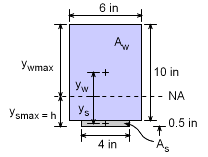0 = ΣEQ = EsQs + EwQw      0 = ( Es/Ew )( ysAs ) + ( Ew/Ew)(ywAw)      0 = 20 (0.25 - h)(0.5)(4) + (5 + 0.5 - h)(6)(10)      0 = 10 - 40h + 330 - 60h      h = 3.40 in Now that the distance h is known, the distance to the center of each material to the NA can be found.       yw= 5.50 - 3.40 = 2.10 in       ys= 0.25 - 3.40 = -3.15 in    With the neutral axis (NA) located, the moment of inertia about the NA for each material can be calculated. Using the basic formula for the moment of inertia for rectangular areas and the parallel axis theorem, gives      Iw= h3 (b/12) + yw2Aw        = 103 (6/12) + 2.12 (6)(10)        = 764.6 in4     Is = h3 (b/12 ) + ys2As        = 0.53 (4/12) + 3.152 (4)(0.5)        = 19.89 in4 The bending stress in each material areas can be determined by using the bending stress equations for a two material composite beam. There are two equations since each material has a different stress.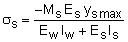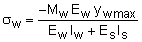The y distance is the maximum distance from the neutral axis for each material section.      ysmax = h = -3.40 in      ywmax = 10 + 0.5 - h = 10 + 0.5 - 3.40 = 7.10 in Substituting values in both the stress equations and using Es/Ew = 20 gives      18,000 = -Ms (-3.40) / [764.6/20 + 19.89]      Ms = 307,700 lb-in = 25,640 lb-ft      -1,200 = -Mw (7.10) / [764.6 + 20 (19.89)]      Mw = 196,500 lb-in = 16,380 lb-ft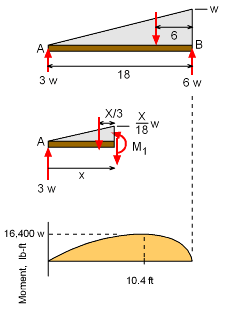Thus, when the moment reaches 16.38 kip-ft, the wood will fail in compression. So what load w will generate a moment of 16,380 lb-ft? To answer this, the moment equation needs to be determined in terms of w. The reaction at A is    ΣMB = 0 = -18 FA + 6 [0.5 w 18]    FA = 3w (assume all units are in ft and kip) Next, find the moment equation     ΣMcut = 0 = M1 + [0.5 x (wx/18)] (x/3) - 3wx     M1 = 3wx - x3w/108 Maximum moment will occur at,     dM1 / dx = 0 = 3w - x2w/36     x = 10.39 Maximum moment in terms of w is      Mmax = 20.78 w To generate a moment of 16,380 lb-ft (wood failure), the loading function w must be      16,380 = 20.78 w      w = 788.3 lb/ft

Practice Homework and Test problems now available in the 'Eng Mechanics' mobile app
Includes over 400 problems with complete detailed solutions.
Available now at the Google Play Store and Apple App Store.